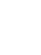# Matematički model površina - UvodPreuzimanje (Download)Ugradnja (Embed) zatvori Ugradi (Embed) izvršnu kopiju ove simulacije Koristi ovaj HTML za ugradnju (embed) izvršne kopije ove simulacije. Možeš mijenjati dimenzije prikaza (širinu i visinu ekrana) mijenjajući "width" i "height" atribute u HTML. Ugradi sliku. Klikom na nju pokretat će se simulacija.
Klikni i započni
Koristi ovaj HTML kod da bi se pojavio ekran sa natpisom "Klikni i započni" ("Click to Run"). Factors Produkti Area Model PhET je podržаn od:i nаstаvnikа kаo što ste Vi!

• Factors
• Produkti
• Area Model
• Množenje
• Partial Products

### Description

Build rectangles of various sizes and relate multiplication to area. Partition a rectangle into two areas to discover the distributive property.

### Primjeri obrazovnih ciljeva

• Recognize that area represents the product of two numbers.
• Develop and justify a strategy that uses the area model to simplify a multiplication problem.
• Represent a multiplication problem as the proportional area of a rectangle.
• Looks for patterns in the total area calculation.

### Standards Alignment

#### Common Core - Math

2.NBT.A.2
Count within 1000; skip-count by 5s, 10s, and 100s.
2.OA.C.4
Use addition to find the total number of objects arranged in rectangular arrays with up to 5 rows and up to 5 columns; write an equation to express the total as a sum of equal addends.
3.MD.C.7
Relate area to the operations of multiplication and addition.
3.MD.C.7c
Use tiling to show in a concrete case that the area of a rectangle with whole-number side lengths a and b + c is the sum of a × b and a × c. Use area models to represent the distributive property in mathematical reasoning.
3.MD.C.7d
Recognize area as additive. Find areas of rectilinear figures by decomposing them into non-overlapping rectangles and adding the areas of the non-overlapping parts, applying this technique to solve real world problems.
3.OA.A.1
Interpret products of whole numbers, e.g., interpret 5 × 7 as the total number of objects in 5 groups of 7 objects each. For example, describe a context in which a total number of objects can be expressed as 5 × 7.
3.OA.A.3
Use multiplication and division within 100 to solve word problems in situations involving equal groups, arrays, and measurement quantities, e.g., by using drawings and equations with a symbol for the unknown number to represent the problem.1
3.OA.B.5
Apply properties of operations as strategies to multiply and divide.2 Examples: If 6 × 4 = 24 is known, then 4 × 6 = 24 is also known. (Commutative property of multiplication.) 3 × 5 × 2 can be found by 3 × 5 = 15, then 15 × 2 = 30, or by 5 × 2 = 10, then 3 × 10 = 30. (Associative property of multiplication.) Knowing that 8 × 5 = 40 and 8 × 2 = 16, one can find 8 × 7 as 8 × (5 + 2) = (8 × 5) + (8 × 2) = 40 + 16 = 56. (Distributive property.)
4.OA.A.1
Interpret a multiplication equation as a comparison, e.g., interpret 35 = 5 × 7 as a statement that 35 is 5 times as many as 7 and 7 times as many as 5. Represent verbal statements of multiplicative comparisons as multiplication equations.
Version 1.2.1

### Teacher-Submitted Activities

Middle School Math Sim AlignmentAmanda McGarry MS (SŠ) Ostalo Matematika
PRIMARIA: Alineación con programas de la SEP México (2011 y 2017)Diana López MS (SŠ)
K-5 (OŠ)
Discuss
Demo
Lab
Guided
DZ
Matematika
Hemija
Astronomija
Fizika
Area Model Intro Simulation Nailah Shah-Taylor K-5 (OŠ) Discuss
Guided
Matematika
Jezik Downloador RunTips
Albanski All shqipModeli i Syprinës: Hyrje
Arapski All العربيةمقدمة مساحة النموذج
Baskijski All EuskaraAzalera-modelorako sarrera
Brazilski Portugalski All português (Brasil)Modelo de Área: Intro
Danski All DanskArealmodel, introduktion
Engleski All EnglishArea Model Introduction
Finski All suomiPinta-alamallin johdanto
Francuski All françaisIntroduction à la modélisation par les aires
Grčki All ΕλληνικάΥπολογισμός εμβαδού - Εισαγωγή
Gujarati All Gujaratiક્ષેત્રફળના મોડેલની પ્રસ્તાવના - નિકુંજ સવાણી દ્વારા
Hindu All हिंदीनमूने का क्षेत्रफल -परिचय
Holandski All NederlandsOppervlakte model inleiding
Italijanski All italianoRappresentazione grafica della moltiplicazione: introduzione
Japanski All 日本語面積の基本
Kazaški All KazakhАуданын табудың негіздері
Kineski - Tradicionalni All 中文 (台灣)Area Model Introduction_面積模型介紹
Kineski - Uprošćen All 中文 (中国)面积模型入门
Korejski All 한국어면적 모형: 소개
Letonski All LatviešuLauka modeļa ievads
Mađarski All magyarTerületmodell: bevezetés
Mongolski All Монгол (Монгол)Талбайн Загварын Танилцуулга
Njemački All DeutschFlächenmodell Einführung
Persijski All فارسیمعرفی مدل مساحت
Poljski All polskiModel pola - wstęp
Portugalski All portuguêsModelo de Área: Introdução
Ruski All русскийОсновы нахождения площади
Sinhaležanski All Sinhaleseවර්ගඵලය ආකෘති හඳුන්වාදීම
Španski All españolModelo de Áreas: Introducción
Španski (Meksiko) All español (México)Modelo de Áreas: Introducción
Španski (Peru) All español (Perú)Modelo de Área. Introducción
Srpski All СрпскиМатематика преко површина-Увод
Švedski All svenskaAreamodellen -Introduktion
Turski All TürkçeAlan Modeline Giriş
Ukrajinski All українськаПлощинна модель. Вступ
Vijetnamski All Tiếng ViệtGiói thiệu Mô hình diện tích

HTML5 sims can run on iPads and Chromebooks, as well as PC, Mac, and Linux systems.

iOS 12+ Safari

Android:
Not officially supported. If you are using the HTML5 sims on Android, we recommend using the latest version of Google Chrome.

Chromebook:
The HTML5 and Flash PhET sims are supported on all Chromebooks.
Chromebook compatible sims

Windows Systems:

Macintosh Systems:

Linux Systems:

Dizajn tim Nezavisne baze i biblioteke Hvala:
• Jonathan Olson (developer)
• Karina Hensberry
• Susan Miller
• Ariel Paul
• Kathy Perkins
• Mariah Hermsmeyer (artwork)
• Diana López Tavares (artwork)
• almond-0.2.9.js
• base64-js-1.2.0.js
• FileSaver-b8054a2.js
• font-awesome-4.5.0
• game-up-camera-1.0.0.js
• he-1.1.1.js
• himalaya-0.2.7.js
• jama-1.0.2
• jquery-2.1.0.js
• lodash-4.17.4.js
• pegjs-0.7.0.js
• seedrandom-2.4.2.js
• text-2.0.12.js
• TextEncoderLite-3c9f6f0.js